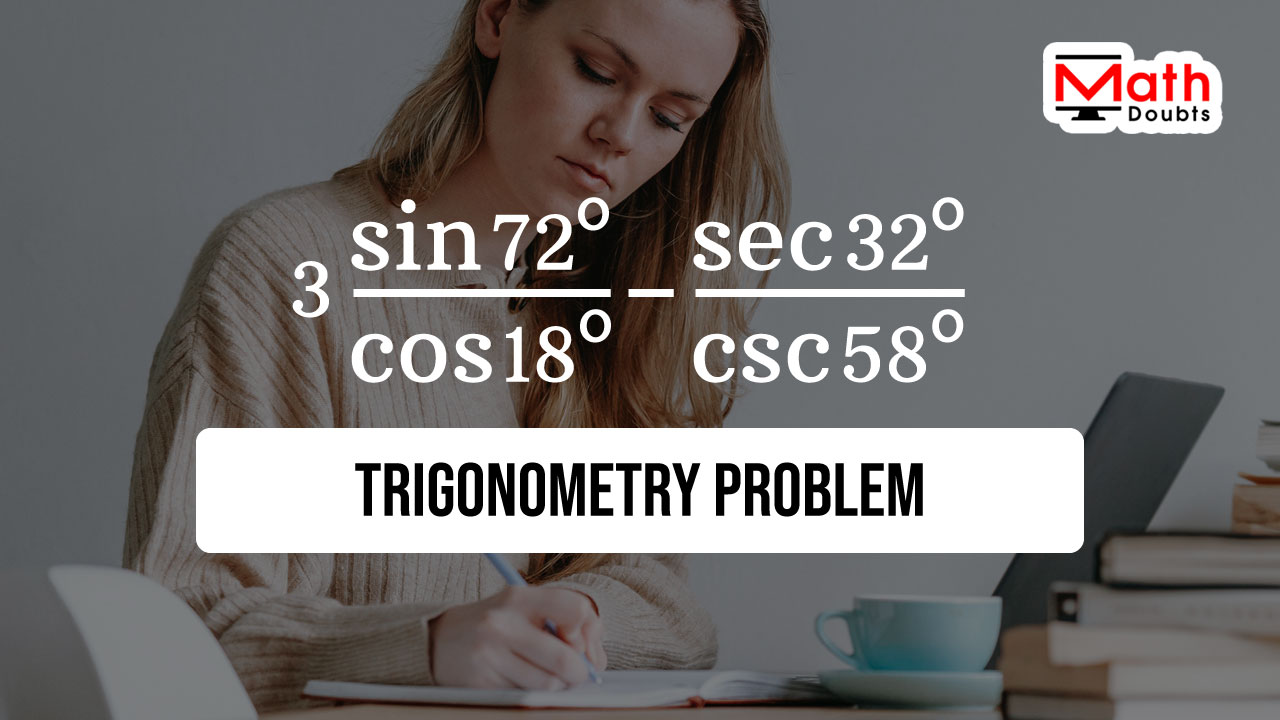# Evaluate $3\dfrac{\sin{72^\circ}}{\cos{18^\circ}}$ $-$ $\dfrac{\sec{32^\circ}}{\csc{58^\circ}}$

Three times sine of angle seventy two degrees divided by cosine of angle eighteen degrees minus secant of angle thirty two degrees divided by cosecant of angle fifty eight degrees is a given trigonometric expression in this trigonometry problem.The angles in sine, cosine, secant and cosecant functions are not standard angles. So, it is hard to remember the values of sine of angle $72^\circ$, cosine of angle $18^\circ$, secant of angle $32^\circ$ and cosecant of angle $58^\circ$. However, the trigonometric expression can be evaluated without substituting their values.

### Calculate the value of the first term

The angles inside the sine and cosine functions in the first term are seventy two and eighteen degrees respectively. The sum of them is a right angle. In other words, $72^\circ +18^\circ \,=\, 90^\circ$. Therefore, the angles of the trigonometric functions in the first term are complementary angles.

$=\,\,\,$ $3 \times \dfrac{\sin{72^\circ}}{\cos{18^\circ}}$ $-$ $\dfrac{\sec{32^\circ}}{\csc{58^\circ}}$

The angle eighteen degrees can be written as the subtraction of angle seventy two degrees from the angle ninety degrees.

$=\,\,\,$ $3 \times \dfrac{\sin{72^\circ}}{\cos{(90^\circ-72^\circ)}}$ $-$ $\dfrac{\sec{32^\circ}}{\csc{58^\circ}}$

According to the cofunction identity of cosine function, the cosine of ninety degrees minus seventy two is equal to the sine of angle seventy two degrees.

$=\,\,\,$ $3 \times \dfrac{\sin{72^\circ}}{\sin{(72^\circ)}}$ $-$ $\dfrac{\sec{32^\circ}}{\csc{58^\circ}}$

It is time to simplify the trigonometric expression in the first term to find its value.

$=\,\,\,$ $3 \times \dfrac{\sin{72^\circ}}{\sin{72^\circ}}$ $-$ $\dfrac{\sec{32^\circ}}{\csc{58^\circ}}$

$=\,\,\,$ $3 \times \dfrac{\cancel{\sin{72^\circ}}}{\cancel{\sin{72^\circ}}}$ $-$ $\dfrac{\sec{32^\circ}}{\csc{58^\circ}}$

$=\,\,\,$ $3 \times 1$ $-$ $\dfrac{\sec{32^\circ}}{\csc{58^\circ}}$

$=\,\,\,$ $3$ $-$ $\dfrac{\sec{32^\circ}}{\csc{58^\circ}}$

### Evaluate the value of the second term

Now, let’s focus on finding the trigonometric expression in the second term. In fact, the trigonometric identities in secant and cosecant functions are not always useful when their use is compared with the use of their reciprocal trigonometric functions. Therefore, it is always better to express secant and cosecant functions in their reciprocal form.

$=\,\,\,$ $3$ $-$ $\dfrac{\sec{32^\circ} \times 1}{\csc{58^\circ}}$

Now, split the second term as a product of two functions as per the multiplication of the fractions.

$=\,\,\,$ $3$ $-$ $\sec{32^\circ}$ $\times$ $\dfrac{1}{\csc{58^\circ}}$

According to the reciprocal identity of cosecant function, the cosecant of angle fifty eight degrees is written as sine of angle fifty eight degrees.

$=\,\,\,$ $3$ $-$ $\sec{32^\circ}$ $\times$ $\sin{58^\circ}$

According to the reciprocal identity of cosine function, the secant of angle thirty two degrees can be expressed as a reciprocal of sine of angle thirty two degrees.

$=\,\,\,$ $3$ $-$ $\dfrac{1}{\cos{32^\circ}}$ $\times$ $\sin{58^\circ}$

Now, multiply both functions as per the multiplication of the fractions.

$=\,\,\,$ $3$ $-$ $\dfrac{1 \times \sin{58^\circ}}{\cos{32^\circ}}$

$=\,\,\,$ $3$ $-$ $\dfrac{\sin{58^\circ}}{\cos{32^\circ}}$

The angles insides the sine and cosine functions are fifty eight and thirty two degrees respectively, and their sum is ninety degrees. It means, $58^\circ+32^\circ \,=\, 90^\circ$. So, the angle fifty eight degrees can be written as the subtraction of thirty two degrees from ninety degrees.

$=\,\,\,$ $3$ $-$ $\dfrac{\sin{(90^\circ-32^\circ)}}{\cos{32^\circ}}$

According to the cofunction identity of sine function, the sine of angle ninety degrees minus thirty two degrees is equal to the cosine of angle thirty two degrees.

$=\,\,\,$ $3$ $-$ $\dfrac{\cos{32^\circ}}{\cos{(32^\circ)}}$

$=\,\,\,$ $3$ $-$ $\dfrac{\cos{32^\circ}}{\cos{32^\circ}}$

$=\,\,\,$ $3$ $-$ $\dfrac{\cancel{\cos{32^\circ}}}{\cancel{\cos{32^\circ}}}$

### Find the value of expression by simplification

The trigonometric expression is successfully simplified as an arithmetic expression and it is time to find its value mathematically.

$=\,\,\,$ $3-1$

$=\,\,\,$ $2$

Latest Math Topics
Jun 26, 2023
Jun 23, 2023

Latest Math Problems
Jul 01, 2023
Jun 25, 2023
###### Math Questions

The math problems with solutions to learn how to solve a problem.

Learn solutions

Practice now

###### Math Videos

The math videos tutorials with visual graphics to learn every concept.

Watch now

###### Subscribe us

Get the latest math updates from the Math Doubts by subscribing us.